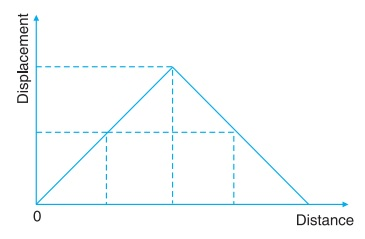# Distance and Displacement

For a moving object two points are significant. One is the point of start or origin where from the object starts its motion and the other is the point where it reaches after certain interval of time. Points of start and destination are connected by a path taken by the object during its motion.

The length of the path followed by object is called distance. There may be a number of paths between the point of start and the point of destination. Hence the object may cover different distances between same point of start and destination. The unit of distance is metre (m) or kilometre (km).

In any motion, the object gets displaced while it changes its position continuously. The change in position of the object is called displacement. Basically, it is the shortest distance between initial and final position of the object.

The path followed by the object between initial and final positions may or may not be straight line. Hence, the length of the path does not always represent the displacement.

• Displacement is smaller or equal to the distance.

• Displacement is equal to distance, if body moves along a straight line path and does not change its direction.

• If a body does not move along a straight line path its displacement is less than the distance.

• Displacement can be zero but distance can not be zero.

• Magnitude of displacement is the minimum distance between final position and initial position.

• Distance is the length of the path followed by the body.

• Distance is path dependent while displacement is position dependent.

### Graphical Representation of Distance and Displacement

Distance and displacement can be shown by graphical representation. To draw a graph, follow the following steps:

1. Analyse the range of variables (maximum and minimum values).
2. Select the suitable scale to represent the data on the graph line adequately.
3. Take independent quantity on x-axis and dependent quantity on y-axis.

Take distance on x-axis and displacement on y-axis. For a motion along a straight line without changing its direction the distance is always equal to the displacement. If you draw the graph, you will find that the graph line is a straight line passing through origin making an angle of 45° with distance axis.Take another situation where an object moves from one position to another and comes back to the same position. In this case the graph will be a straight line making an angle of 45° with distance axis up to its maximum value and then comes to zero.If graph is a straight line making an angle of 45° with x-axis or y-axis, the motion is straight line motion and distance is equal to the displacement.

For same value of displacement, the distance traveled can be different. If graph line does not make an angle of 45° with x-axis or y-axis, the motion will not be straight line motion.

When an object moves along a circular path, the maximum displacement is equal to the diameter of the circular path and the distance traveled by object keeps on increasing with time.